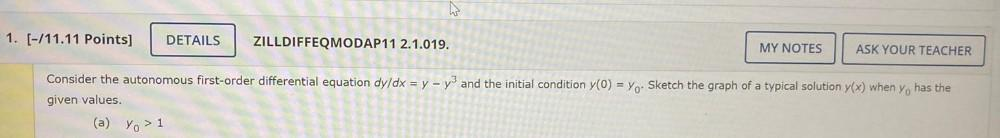Question:

# Consider the autonomous first-order differential equation dy/dx = y - y³ and the initial condition y(0) = y₀. Sketch the graph of a typical solution y(x) when y₀, has the given values. (a) y₀ > 1Consider the autonomous first-order differential equation dy/dx = y - y³ and the initial condition y(0) = y₀. Sketch the graph of a typical solution y(x) when y₀, has the given values. (a) y₀ > 1